AP State Syllabus AP Board 9th Class Maths Solutions Chapter 5 Co-Ordinate Geometry Ex 5.3 Textbook Questions and Answers.

## AP State Syllabus 9th Class Maths Solutions 5th Lesson Co-Ordinate Geometry Exercise 5.3

Question 1.
Plot the following points on the Cartesian plane whose x, y co-ordinates are given.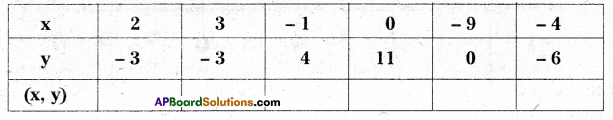Solution: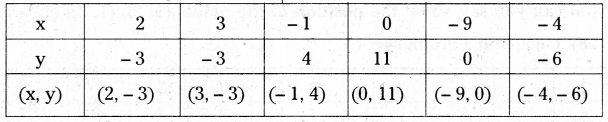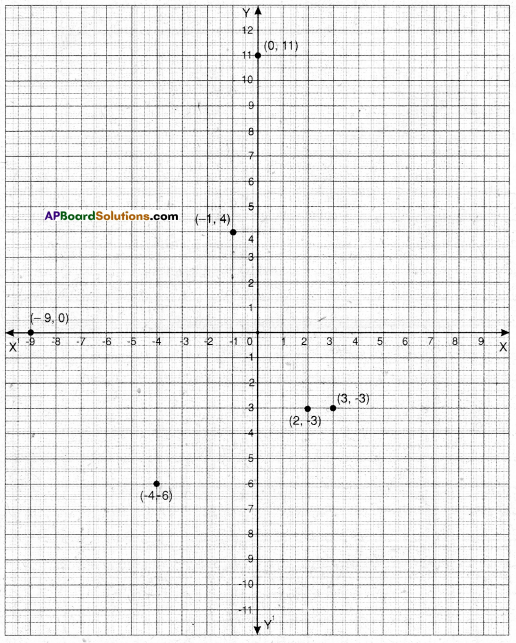Question 2.
Are the positions of (5, -8) and (-8, 5) Is same ? JustIfy your answer.
Solution:
The positions of (5, -8) and (-8, 5) are not same. They are two distinct points. (5, -8) lies at a distance of 5 units from Y – axis and 8 units from X – axis on down side of the origin. So it lies in Q4. Where as (- 8, 5) lies in Q2. The point is at a distance of 8 units from Y – axis on left side of the origin and 5 units from X – axis.

Question 3.
What can you say about the position of the points (1, 2), (1, 3), (1, – 4), (1, 0) and (1, 8), Locate on a graph sheet.
Solution: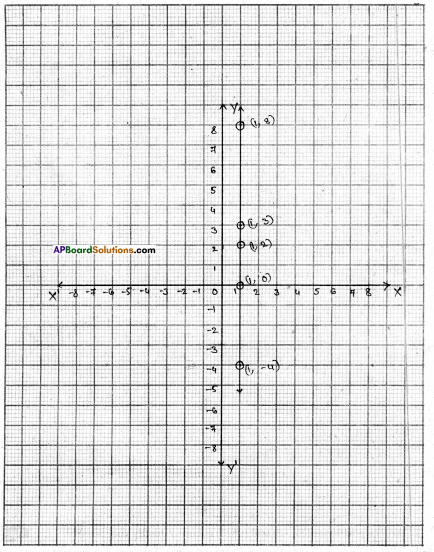All the given points lie on a line parallel to Y-axis at a distance of 1 cm.

Question 4.
What can you say about the position of the points (5, 4), (8, 4), (3, 4), (0, 4), (-4, 4), (-2,4)? Locate the points on a graph sheet and justify your answer.
Solution:
The points (5, 4), (8, 4), (3, 4), (0, 4), (- 4, 4) and (- 2, 4) all lie on a line parallel to X-axis at a distance of 4-units from it.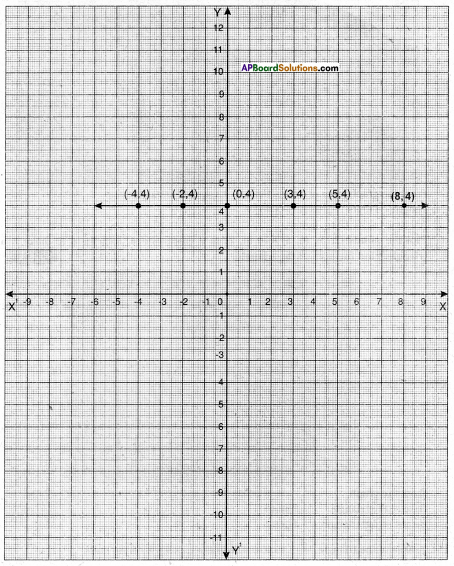Question 5.
Plot the points (0, 3), (4, 3), (3, 4) (4, 0) in graph sheet. Join the points with straight lines to make a rectangle. Find the area of the rectangle.
Solution: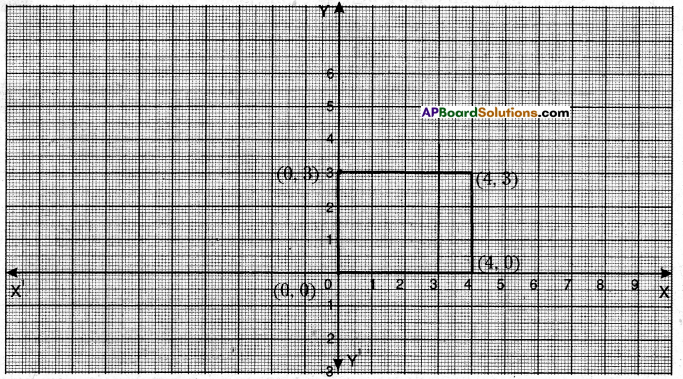From the graph, area of the rectangle =12 square units (OR)
Length = 4 units ; breadth = 3 units
A = l/b = 4 × 3 = 12 sq. units.

Question 6.
Plot the points (2, 3), (6, 3) and (4, 7) in a graph sheet. Join them to make it a triangle. Find the area of the triangle.
Solution: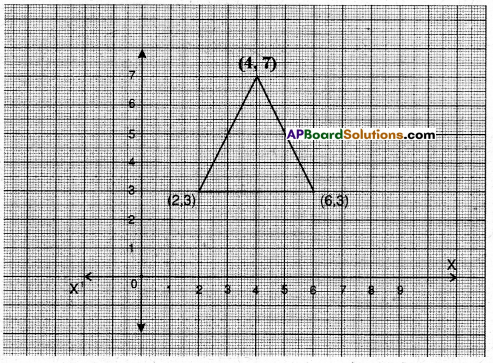From the graph
Base of the triangle = 4 units
Height of the triangle = 4 units
∴ Area of the triangle = $$\frac{1}{2}$$ × base × height = $$\frac{1}{2}$$ × 4 × 4 = 8sq. units.Question 7.
Plot at least six points in a graph sheet, each having the sum of its co-ordinates equal to 5. [Hint: (- 2, 7), (1, 4)………….]
Solution:
Given that (x co-ordinate) + (y co-ordinate) = 5
Let the points be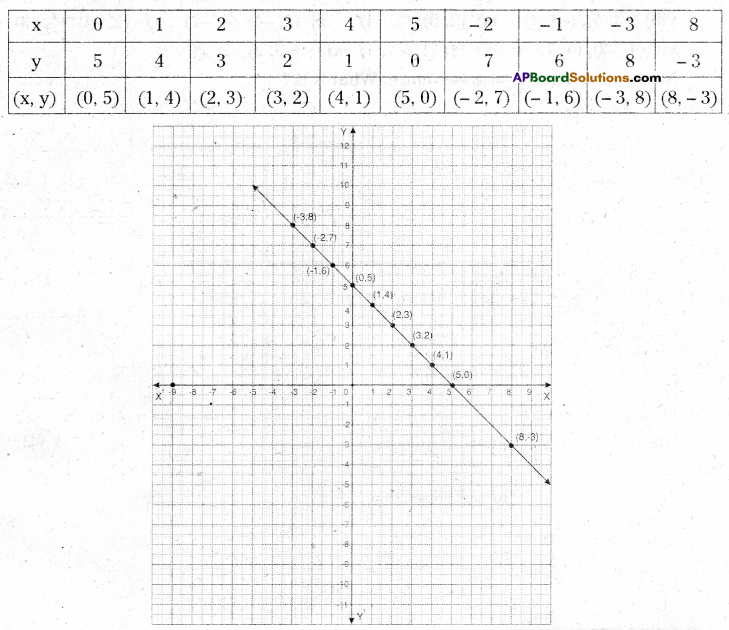Question 8.
Look at the graph. Write the co-ordinates of the points
A, B, C, D, E, F. G. H, 1, J, K, L, M, N, O, P and Q.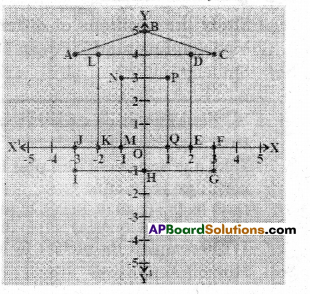Solution:
A(- 3, 4) ; B(0, 5) : C (3, 4) ; D (2, 4) ; E (2, 0) ;
F (3, 0) ; G (3, – 1) ; H (0, – 1) ; I (- 3, – 1) ;
J (- 3, 0) ; K (- 2, 0) ; L (- 2, 4) ; M (- 1, 0) ;
N (-1, 3); O (0, 0) ; P (1. 3) and Q (1, 0)Question 9.
In a graph sheet plot each pair of points, join them by line segments.
i) (2, 5), (4, 7)
ii) (-3, 5) (-1,7)
iii) (-3, -4), (2, -4)
iv) (-3, -5) (2, -5)
v) (4, -2), (4, -3)
vi) (-2, 4), (-2, 3)
vii) (-2, 1), (-2, 0)
Now join the following pairs of points by straight line segments, in the same graph.
viii) (-3, 5), (-3, 4)
ix) (2, 5), (2, -4)
x) (2, -4), (4, -2)
xi) (2, -4), (4, -3)
xii) (4, -2), (4, 7)
xiii) (4, 7), (-1, 7)
xiv) (-3, 2), (2, 2)
Now you will get a surprise figure. What is it ?
Solution: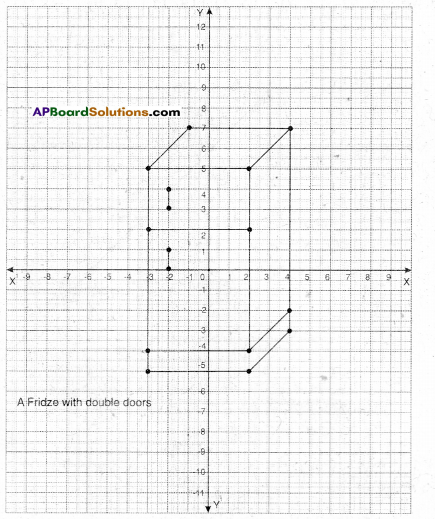AP Board 9th Class Maths Solutions